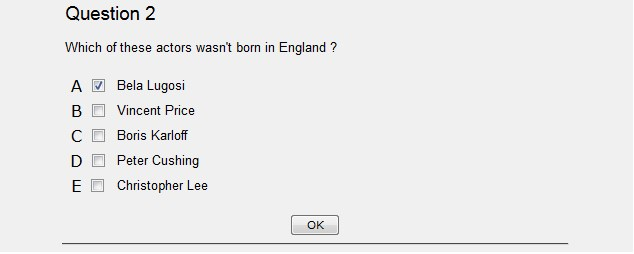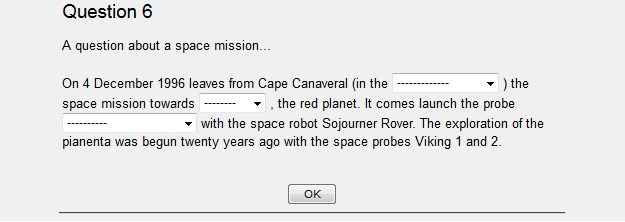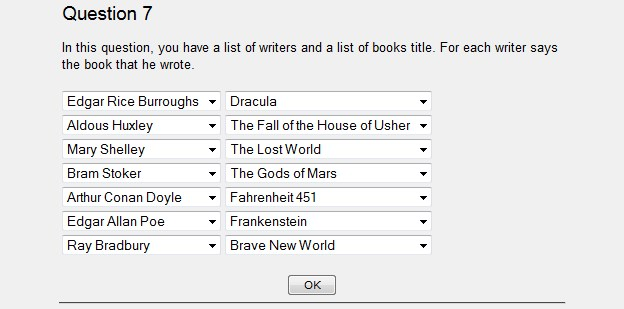With QuizFaber, you can create the following categories of quiz:

#### Multiple choice questions (wrong or right type)

A question can have more than one possible answer. Each answer can be correct or wrong but only one is the correct answer.
Below an example of multiple choice question:If you need a question with more than one right answers, you must use the "Multiple answers" type, as explained below.

#### Multiple answers questions (wrong or right type)

A question can have more than one possible answer. Each answer can be correct or wrong (it is admitted that a question can have more than one correct answer but not none: at least one correct must be inserted).
In that case the student's answer will be considered as correct only if he or she has clicked on the correct answers and only on the correct answers.
Consequently in a question with two possible correct answers, if a students clicks on a wrong answer, he (or she) will score negatively even if he (or she) found the second right answer.
Below an example of multiple answer question:#### True or false questions

The question starts with a text that exposes the content of the question and is followed by a series of statements, each of which can be true or false.
The student's score will depend on the proportion of correct statements he (or she) found out.
Unlike in the multiple answer questions, where an estimated question can be evaluated positively or negatively, a question with true or false can be estimated as partially correct or wrong (the relationship between number of statements guessed out of the total number of proposed affirmations represents the percentage of exact answers: this number will be used in the computation of the final mark).
Below an example of True of false question:It is the type of question in which the student can answer freely, writing the own answer. In particular, in the quiz generated, a text box (empty) appear under the question and the student can write its answer.
Obviously, such an answer cannot be automatically corrected (not yet at least, they might be when computers become intelligent!) For this reason it must always be kept in mind that the assigned final mark at the end of quiz is the mark without the questions with open answers.
The teacher must subsequently mark such answers himself after printing it.
Below an example of open answer question:#### Gap filling exercises

It is a question constituted of a text prepared by the teacher who chooses the words that will be omitted in the quiz. An empty space will appear in their place (a text box in an HTML page or a white space on paper) where the student will have to insert the hidden word.
The mark will depend on the percentage of words correctly inserted by the student. ( the same as the questions with true or false answers).
Naturally it is possible to omit not only a single word but an entire phrase or even sentence, that however increases the difficulty for the student to answer correctly because he must write the exact sentence. Consequently an additional space, a comma, or a simple mistake will entail a negative evaluation.
Below an example of gap filling exercises:Another example of gap filling exercises, where empty text box are replaced by a drop-down list:#### Questions with matching words

The question introduces two distinguished groups of words places on two columns. The student will have to correlate each word of the first group with one of the second.
Below an example of question with matching words:Go to top